WEBINAR
Numerical Analysis of Magnetic Pulse Welding Process
Thursday, October 5, 2023
Time
SESSION 1
SESSION 2
CEST (GMT +2)
03:00 PM
08:00 PM
EDT (GMT -4)
09:00 AM
02:00 PM
HOME / Applications / No-Load and Load Analyses of a Permanent Magnet Axial Flux Generator

# No-Load and Load Analyses of a Permanent Magnet Axial Flux Generator

Used Tools:## Introduction

In this application note, a PM magnet axial flux generator is studied under load and no-load conditions. To perform this analysis we used EMS coupled with SOLIDWORKS Motion. Results, including back emf, short circuit response, current and voltage waveforms at different loads, eddy and iron losses are computed using EMS for SOLIDWORKS. Winding parameters are also calculated leading to a full performance analysis of the machine.

The simulated axial flux permanent magnet machine is shown in Figure 1. It is a double-sided rotor coreless axial flux generator with 24 poles (made from N42 permanent magnets). This kind of generator can be used for transportation applications as well as for energy production.
(More details about axial flux machine can be found in the video below this application or by visiting these links:1 ,2)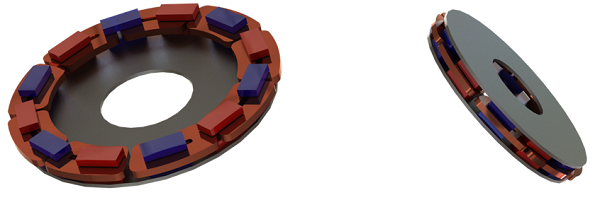Figure 1 - The studied axial flux generator

## Simulation and Results

During the no load analysis, the back emf results are computed for each phase and for different rotor speeds. Figures 2a) and 2b) show the 3phases back emf results at 1200RPM and 15000RPM, respectively. The computed back emf represents a balanced system with a frequency. Of ~150Hz. The peak value of the signal is around 36V at 1200RPM while it is around 45V at 1500 RPM.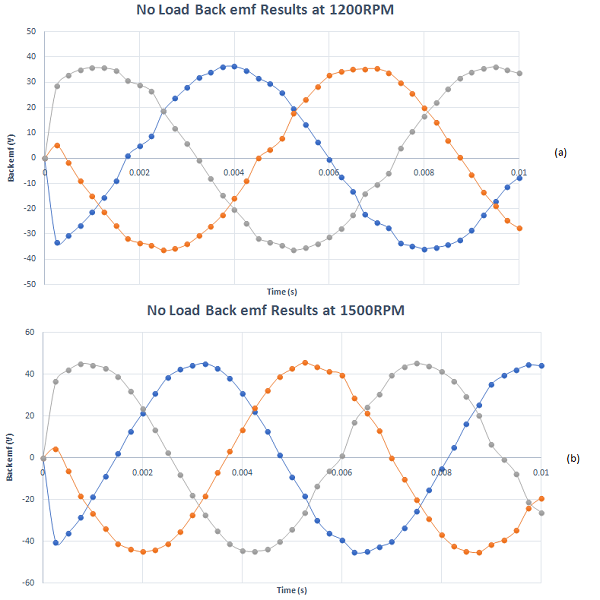Figure 2 - No-Load back emf results, a) at 1200RPM, b) at 1500RPM

The back emf produced by the generator increases with the rotor speed. Figure 3 demonstrates the linear dependency between the output voltage and speed.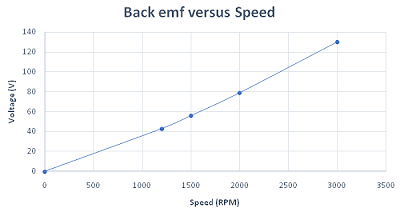Figure 3 - Back emf results versus speed

The speed is not the only factor that affects the generator output voltage. The magnet type and more precisely the magnet remanence has great impact on the back emf of the generator. As can be seen in Figures 4a) 4b) and 4c), the back emf varies with different magnet types. It is minimum when using ceramic magnets and maximum when neodymium magnets are utilized. This is due to the low and high remanence characterizing respectively ceramic and neodymium magnets i.e Br of ceramic grade 1 is 0.22T while Br of N55 is 1.47T.

As concluded from Figure 4, the generator back emf increases with speed. Hence, low remanence magnet can be used at high speed operation to avoid the risk of overvoltage.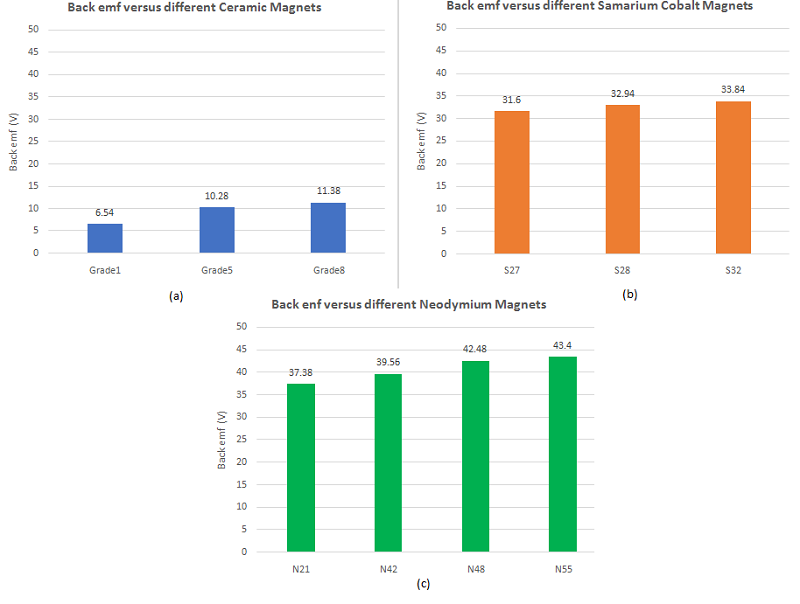Figure 4 - back emf results, a) ceramic magnets, b) samarium cobalt magnets, c) neodymium magnets

### 2- Short circuit Response

Short circuit analysis is important when studying an electrical machine in general. It is conducted to predict the high current produced in the electrical machine when a short circuit occurs. Therefore, the short circuit response of the 3phase axial flux generator was studied. The simulated short circuit schematic, which is performed using EMS circuit simulator, is shown in Figure 5. Each winding represents one phase.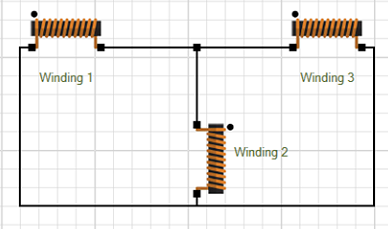Figure 5 -  Short circuit schematic created by EMS circuit simulator

Figure 6 illustrates the generator currents at the short circuit condition while Figure 7 contains the generator currents at normal conditions. It can be seen that the short circuit currents are almost 10 times bigger than normal operation currents. This helps to calculate the maximum current tolerated by protective fuses.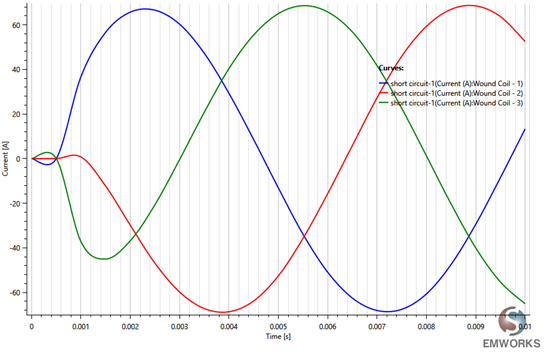Figure 6 - Short circuit currents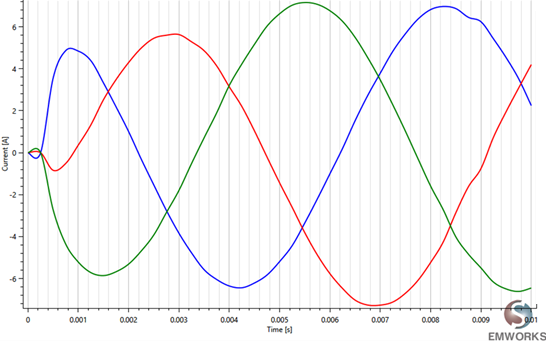Figure 7 - Normal operation currents

The load test of the axial flux generator is performed for different load types using EMS circuit simulator. Figures 8a), 8b) and 8c) contains the different simulated circuits. It includes resistive load, RC load, and RLC load. All are connected in a star connection.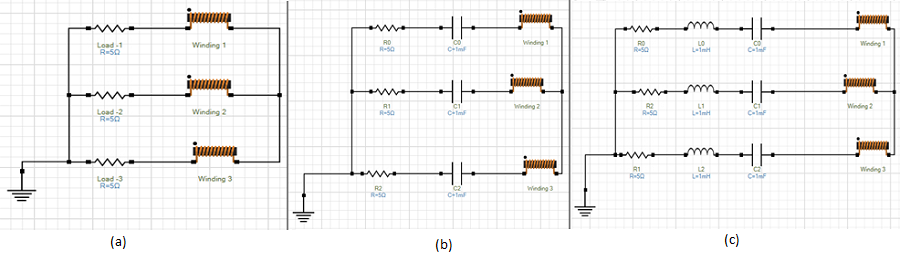The current and voltage results for each load type are computed and plotted in Figures 9a), 9b) and 9c). They have same phase shift in case of resistive load while a slight delay in the other loads.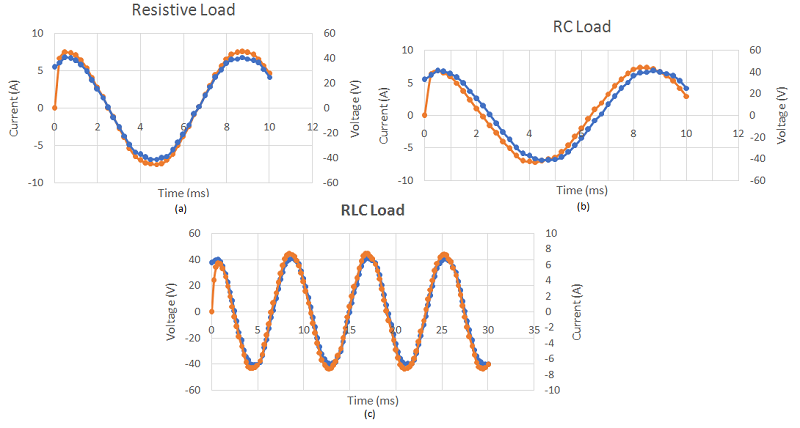#### Electromagnetic losses

Under the load operation, important electromagnetic losses will be generated. this can affect the efficiency of the machine and lead to additional costs of maintenance. Hence, those quantities should be studied carefully during the design of any electrical machines including motors and generators. Eddy, iron and copper losses are predicted by EMS. Winding losses are shown in Figure 10 while the coreloss results are plotted in figure 11. The coreloss is high because of the relatively high frequency voltage generated by this machine.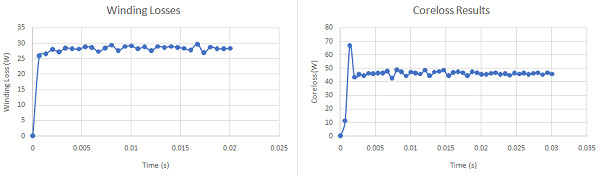Figure 10 - Winding losses                                                     Figure 11 - Coreloss results

In case the machine ferromagnetic cores are not laminated and made of solid bodies, significant eddy currents are expected. Hence, they should be studied separately from coreloss calculation. Figure 12 demonstrates the evolution of the eddy loss in the rotor cores versus time while an animation of the eddy currents mapping is shown in Figure 13.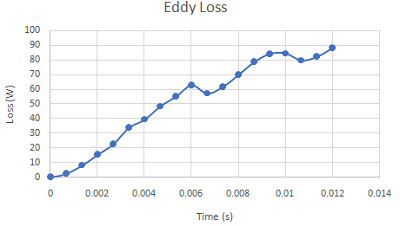Figure 12 - Eddy loss results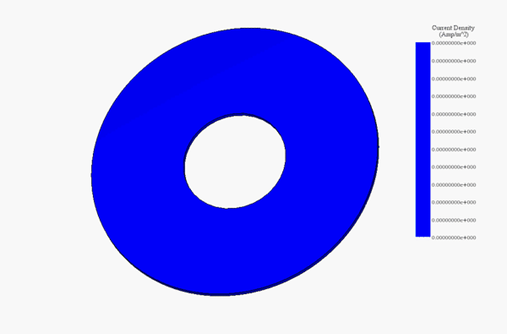Figure 13 - Eddy current distribution versus time

## Summary

In this article, EMS for SOLIDWORKS was used to study the permanent magnet axial flux generator under both load and no-load conditions. The simulation was done by coupling to both Motion and Circuits, which are available inside EMS for SOLIDWORKS as add-in products.

## Videos